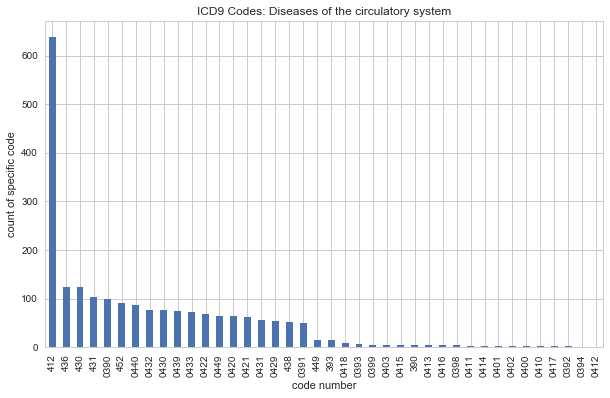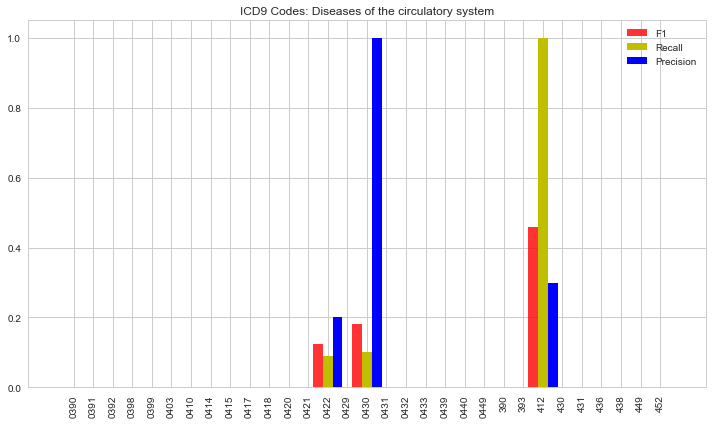# Predict Specific Claims in Medicare Data

Standard

cms-blog

## Introduction¶

Whenever I’m faced with a machine learning task, my goal on day 1 is to build an initial model. The model will without a doubt need to be tuned in days or even weeks after, but it’s good to have a starting point. In the project below, I timeboxed a machine learning inital model of about 4 hours to see how far I get along with some initial results.

## Problem Statement¶

A peer of mine in my Master’s program mentioned there is publicly available Medicare CMS data. I have very little knowledge of healthcare data, but thought I’d explore the data and see if there was an aspect that could be useful in buidling a model to make predictions.

The data:

• 2008 claims outpatient data (used this, only 1 of 20 available samples, still about 1.1 million rows of claims data)
• 2008 beneficiary data (used this)
• 2008 claims inpatient data (did not use this due to initial time constraint)
• 2008 presciption data (did not use this due to initial time constraint)

I identified one useful infomation to build a model on: Predict a medicare claim specific to ICD9 codes relating to diseases of circulatory system (this makes up about 11% of claims).

## Steps included in this project¶

• getting the data
• exploring the data
• identifying potential useful features
• transforming the data
• data exploration
• dimensionality reduction
• selectling initial models
• evaluating initial models
• summarizing

## Useful imports and settings¶

In :
import datetime
import numpy as np
import pandas as pd
from sklearn import linear_model, ensemble, decomposition
from sklearn.preprocessing import MinMaxScaler, Imputer
from sklearn.cross_validation import KFold, cross_val_score
from sklearn.metrics import classification_report, confusion_matrix, precision_recall_curve, recall_score
import matplotlib.pyplot as plt
import seaborn as sns
import warnings

# Settings for plots
%matplotlib inline
plt.rcParams['figure.figsize'] = (10.0, 6.0)
sns.set_style('whitegrid')

pd.options.display.max_columns = None

def warn(*args, **kwargs):
pass
warnings.warn = warn


## Read in data, convert columns, join datasets¶

In :
dateparse = lambda x: pd.datetime.strptime(x, '%Y%m%d')

,parse_dates=, date_parser=dateparse)

# calc age from birthdate
date_2009 = pd.datetime.strptime('20090101', '%Y%m%d')
df_beneficiary1['AGE'] = (date_2009 - df_beneficiary1['BENE_BIRTH_DT']).astype('<m8[Y]')

# join data sets
df_joined = pd.merge(df_outpatient_claims1, df_beneficiary1, left_on='DESYNPUF_ID', right_on='DESYNPUF_ID', how='inner')

print(df_joined.shape)
df_joined.describe()

(790790, 108)

Out:
CLM_ID SEGMENT CLM_FROM_DT CLM_THRU_DT CLM_PMT_AMT NCH_PRMRY_PYR_CLM_PD_AMT AT_PHYSN_NPI OP_PHYSN_NPI OT_PHYSN_NPI NCH_BENE_BLOOD_DDCTBL_LBLTY_AM ICD9_PRCDR_CD_1 NCH_BENE_PTB_DDCTBL_AMT NCH_BENE_PTB_COINSRNC_AMT HCPCS_CD_45 BENE_DEATH_DT BENE_SEX_IDENT_CD BENE_RACE_CD SP_STATE_CODE BENE_COUNTY_CD BENE_HI_CVRAGE_TOT_MONS BENE_SMI_CVRAGE_TOT_MONS BENE_HMO_CVRAGE_TOT_MONS PLAN_CVRG_MOS_NUM SP_ALZHDMTA SP_CHF SP_CHRNKIDN SP_CNCR SP_COPD SP_DEPRESSN SP_DIABETES SP_ISCHMCHT SP_OSTEOPRS SP_RA_OA SP_STRKETIA MEDREIMB_IP BENRES_IP PPPYMT_IP MEDREIMB_OP BENRES_OP PPPYMT_OP MEDREIMB_CAR BENRES_CAR PPPYMT_CAR AGE
count 7.907900e+05 790790.000000 7.795370e+05 7.795370e+05 790790.000000 790790.000000 7.730000e+05 1.344330e+05 2.576660e+05 790790.000000 200.000000 790790.000000 790790.000000 0.0 2.086000e+03 790790.000000 790790.000000 790790.000000 790790.000000 790790.000000 790790.000000 790790.000000 790790.000000 790790.000000 790790.000000 790790.000000 790790.000000 790790.000000 790790.000000 790790.000000 790790.000000 790790.000000 790790.000000 790790.000000 790790.000000 790790.000000 790790.000000 790790.000000 790790.000000 790790.000000 790790.000000 790790.000000 790790.000000 790790.000000
mean 5.425026e+14 1.014230 2.008925e+07 2.008929e+07 283.924569 10.239760 4.975733e+09 4.947815e+09 4.904993e+09 0.012898 5535.690000 2.825466 83.845876 NaN 2.008088e+07 1.577348 1.250675 25.668658 377.350066 11.681684 11.530626 2.681388 7.501712 1.653897 1.489232 1.668855 1.872031 1.729455 1.608191 1.348555 1.304843 1.708268 1.721176 1.911604 4657.439687 526.227770 198.064594 1943.823152 593.896534 66.611920 2240.245678 620.647972 34.333034 72.318409
std 2.858482e+11 0.118438 7.475135e+03 7.471623e+03 571.392794 234.668372 2.874373e+09 2.890483e+09 2.889353e+09 2.315506 3164.871486 15.596522 178.759708 NaN 2.580152e+02 0.493981 0.708571 15.140097 266.921315 1.769500 2.121507 4.914490 5.714692 0.475727 0.499884 0.470625 0.334056 0.444241 0.488155 0.476513 0.460341 0.454560 0.448421 0.283871 12205.547142 1289.921983 2646.498161 3849.941441 1055.746848 597.738709 2228.993683 580.428308 121.383274 13.006691
min 5.420123e+14 1.000000 2.007121e+07 2.008010e+07 -100.000000 0.000000 1.024080e+05 3.258650e+05 1.024080e+05 0.000000 61.000000 0.000000 0.000000 NaN 2.008010e+07 1.000000 1.000000 1.000000 0.000000 0.000000 0.000000 0.000000 0.000000 1.000000 1.000000 1.000000 1.000000 1.000000 1.000000 1.000000 1.000000 1.000000 1.000000 1.000000 -3000.000000 0.000000 0.000000 -90.000000 0.000000 0.000000 0.000000 0.000000 0.000000 25.000000
25% 5.422523e+14 1.000000 2.008092e+07 2.008093e+07 40.000000 0.000000 2.521572e+09 2.474254e+09 2.443058e+09 0.000000 3771.750000 0.000000 0.000000 NaN 2.008070e+07 1.000000 1.000000 11.000000 141.000000 12.000000 12.000000 0.000000 0.000000 1.000000 1.000000 1.000000 2.000000 1.000000 1.000000 1.000000 1.000000 1.000000 1.000000 2.000000 0.000000 0.000000 0.000000 240.000000 60.000000 0.000000 760.000000 220.000000 0.000000 67.000000
50% 5.425023e+14 1.000000 2.009043e+07 2.009043e+07 80.000000 0.000000 4.904972e+09 4.870758e+09 4.774818e+09 0.000000 4533.500000 0.000000 20.000000 NaN 2.008090e+07 2.000000 1.000000 25.000000 350.000000 12.000000 12.000000 0.000000 12.000000 2.000000 1.000000 2.000000 2.000000 2.000000 2.000000 1.000000 1.000000 2.000000 2.000000 2.000000 0.000000 0.000000 0.000000 810.000000 260.000000 0.000000 1570.000000 460.000000 0.000000 73.000000
75% 5.427523e+14 1.000000 2.009120e+07 2.009121e+07 200.000000 0.000000 7.501324e+09 7.485573e+09 7.491945e+09 0.000000 8745.250000 0.000000 70.000000 NaN 2.008110e+07 2.000000 1.000000 38.000000 570.000000 12.000000 12.000000 0.000000 12.000000 2.000000 2.000000 2.000000 2.000000 2.000000 2.000000 2.000000 2.000000 2.000000 2.000000 2.000000 3000.000000 1024.000000 0.000000 2030.000000 680.000000 0.000000 3080.000000 860.000000 10.000000 81.000000
max 5.429923e+14 2.000000 2.010123e+07 2.010123e+07 3300.000000 14000.000000 9.999886e+09 9.999615e+09 9.999470e+09 800.000000 9961.000000 200.000000 1100.000000 NaN 2.008120e+07 2.000000 5.000000 54.000000 999.000000 12.000000 12.000000 12.000000 12.000000 2.000000 2.000000 2.000000 2.000000 2.000000 2.000000 2.000000 2.000000 2.000000 2.000000 2.000000 164220.000000 53096.000000 68000.000000 50020.000000 12450.000000 14400.000000 21160.000000 5260.000000 2040.000000 100.000000

## Identify Features from Beneficiary data (just grabbed them all to start)¶

In :
features = ['AGE', 'BENE_RACE_CD', 'BENE_COUNTY_CD', 'BENE_ESRD_IND', 'BENE_HI_CVRAGE_TOT_MONS', 'BENE_SMI_CVRAGE_TOT_MONS'
, 'BENE_HMO_CVRAGE_TOT_MONS', 'PLAN_CVRG_MOS_NUM','SP_ALZHDMTA'
, 'SP_CHF', 'SP_CHRNKIDN', 'SP_CNCR', 'SP_COPD', 'SP_DEPRESSN', 'SP_DIABETES', 'SP_ISCHMCHT'
, 'SP_OSTEOPRS', 'SP_RA_OA', 'SP_STRKETIA']

# The name of the column for the output varaible.
target = 'ICD9_DGNS_CD_1'


## Group Target ICD9 codes from Claims data (chose Circulatory System Diseases – which is 1 of 17 ICD9 groupings)¶

In :
# 390 ‐ 459 Diseases of the circulatory system
df_joined_circ = df_joined.where(pd.to_numeric(df_joined['ICD9_DGNS_CD_1'], errors='coerce')>=390)
df_joined_circ = df_joined_circ.where(pd.to_numeric(df_joined_circ['ICD9_DGNS_CD_1'], errors='coerce')<=459)

# reduce data for only circulatory codes
df_null_target = df_joined_circ['ICD9_DGNS_CD_1'].notnull()
df_joined_cleaned = df_joined_circ.loc[df_null_target]
print('shape of data', df_joined_cleaned.shape)

shape of data (2144, 108)

In :
df_joined_cleaned[target].value_counts().plot(kind='bar')
plt.xlabel('code number')
plt.ylabel('count of specific code')
plt.title('ICD9 Codes: Diseases of the circulatory system')
print('ICD9 code 412 makes up about', df_joined_cleaned[target].value_counts()/df_joined_cleaned.shape, 'of the data')

ICD9 code 412 makes up about 0.298041044776 of the data## Before running any model, more preprossing needed to convert text to numbers, and dealing with NaN¶

In :
# replace Y and N
df_joined_cleaned['BENE_ESRD_IND'] = df_joined_cleaned['BENE_ESRD_IND'].astype(str)
df_joined_cleaned.BENE_ESRD_IND.replace(['Y', '0'], [1, 0], inplace=True)

# replace NaN with median, mean, most_frequent
imp = Imputer(missing_values='NaN', strategy='most_frequent', axis=0)
imp.fit(df_joined_cleaned[features])
df_joined_cleaned[features] = imp.transform(df_joined_cleaned[features])


## Split data into train and test¶

In :
from sklearn.cross_validation import train_test_split

x = df_joined_cleaned[features]
y = df_joined_cleaned[target]

# Divide the data into a training and a test set.
random_state = 0  # Fixed so that everybody has got the same split
test_set_fraction = 0.2
x_train, x_test, y_train, y_test = train_test_split(x, y, test_size=test_set_fraction,
random_state=random_state)

print('Size of training set: {}'.format(len(x_train)))
print('Size of test set: {}'.format(len(x_test)))

Size of training set: 1715
Size of test set: 429


## Running two algorithms: Random Forest and Logistic Regression¶

In :
rf = ensemble.RandomForestClassifier(random_state=15) # set seed
rf.fit(x_train, y_train)
print('random forest model score', rf.score(x_test,y_test))

lm = linear_model.LogisticRegression()
lm.fit(x_train,y_train)
print('logistic regression model score', lm.score(x_test,y_test))

y_pred = lm.predict(x_test)

random forest model score 0.20979020979
logistic regression model score 0.286713286713


## Dimensionality Reduction with PCA¶

In :
pca = decomposition.PCA(n_components=9)
print('original shape prior to PCA', x_train.shape)
x_train_new = pca.fit_transform(x_train)
x_test_new = pca.transform(x_test)
print('new shape after to PCA', x_train_new.shape)

original shape prior to PCA (1715, 19)
new shape after to PCA (1715, 9)


## Run Algorithms again with reduced features thanks to PCA¶

In :
rf = ensemble.RandomForestClassifier(random_state=15) # set seed
rf.fit(x_train_new, y_train)
print('random forest model score', rf.score(x_test_new, y_test))

lm = linear_model.LogisticRegression()
lm.fit(x_train_new, y_train)
print('logistic regression model score', lm.score(x_test_new, y_test))

print('After dimensionality reduction, our performance increased on Logistic Regression, and performed better than Random Forest')

random forest model score 0.198135198135
logistic regression model score 0.298368298368
After dimensionality reduction, our performance increased on Logistic Regression, and performed better than Random Forest


## Plotting Results¶

In :
# get class list for chart
def class_classification_report(cr, title='Classification report ', with_avg_total=False, cmap=plt.cm.Blues):

lines = cr.split('\n')
classes = []

for line in lines[2 : (len(lines) - 3)]:
t = line.split()
classes.append(t)
return classes

y_pred = lm.predict(x_test_new)
y_label = class_classification_report((classification_report(y_test, y_pred)))

In :
from sklearn.metrics import recall_score, f1_score, precision_score

print('Recall = ', recall_score(y_test, y_pred, average='weighted'))
print('Precision = ', precision_score(y_test, y_pred, average='weighted'))
print('F1 = ', f1_score(y_test, y_pred, average='weighted'))

Recall =  0.298368298368
Precision =  0.116132524663
F1 =  0.142505332535

In :
# plot Precision, Recall, F1
n_groups = len(f1_score(y_test, y_pred, average=None))
index = np.arange(n_groups)

width = 0.5
fig, ax = plt.subplots()
rects1 = ax.bar(index, f1_score(y_test, y_pred, average=None)
, width, alpha=0.8, color='r')
rects2 = ax.bar(index + width, recall_score(y_test, y_pred, average=None)
, width, color='y')
rects3 = ax.bar(index + width * 2, precision_score(y_test, y_pred, average=None)
, width, color='b')
ax.legend((rects1, rects2, rects3), ('F1', 'Recall', 'Precision'))
plt.xticks(index + width, np.sort(y_label), rotation=90)
plt.title('ICD9 Codes: Diseases of the circulatory system')
plt.tight_layout()
plt.show()## Interpreting Model Results¶

Of the 40 ICD9 codes representing Circulatory diseases, my model only produced predicted for 0422, 0430, and 412, which isn’t ideal, but those three codes make us 37% of my training data. Above, I plotted, recall, precision, and f1 scores. I like using f1 score as it’s really a balance of recall & precision (what portion of true-positives is your model getting and how good it is at predicting true-positives). At this point, much more investigating the data and tweaking the models is needed to improve performance. Gaining domain knowledge is this field would certaining help too!

The data is unbalanced, and if I would have just guessed code 412 for all instances, my Recall rate would have increase, but then my Precision and f1 would have dropped.

## Final Thoughts¶

This was a “quick and dirty” model building exercise, which didn’t produce great results, but is a good starting point. Rarely are you going to get great results with limited amount of work.

Overall, there is a some opportunity here, but would take many more iterations of model tuning. I would recommend bringing in the Drug Prescription data source, along with a couple more years of claims data so health trends by patient could be leveraged.